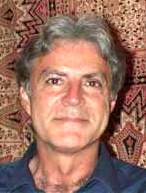Enter the content which will be displayed in sticky bar

# MemberDr. Gianfranco Spavieri
local time: 2022-05-17 06:08 (-04:00 )
Dr. Gianfranco Spavieri (Abstracts)
Titles Abstracts Details

The introduction of the ZPF leads to a probability density p0(v) (where v is the electron speed) similar to the Fermi-Dirac distribution, and to a correlation function CG(? ) of the conductance G, which, in a small, unique v interval ?v (where the electrons are at the threshold of runaways) decays as ??" with 0:003 ? " ? 0:007. The corresponding power spectral density turns out to be SG(f) = G2?"N?1(2??m)"f"?1, where f is the frequency, N the total number of electrons in the considered sample, ?m the information transmission time, and ?" a dimensionless quantity depending on electron number density N. For the purest semiconductors, ?" that turns out to be in excellent agreement with the experimental data vs N. The above result also holds for a ?nite sample because the electron di?usion in the small ?v is much more rapid than the drift velocity.

• [Updated 5 years ago]

The introduction of the ZPF leads to a probability density p0(v) (where v is the electron speed) similar to the Fermi-Dirac distribution, and to a correlation function CG(? ) of the conductance G, which, in a small, unique v interval ?v (where the electrons are at the threshold of runaways) decays as ??" with 0:003 ? " ? 0:007. The corresponding power spectral density turns out to be SG(f) = G2?"N?1(2??m)"f"?1, where f is the frequency, N the total number of electrons in the considered sample, ?m the information transmission time, and ?" a dimensionless quantity depending on electron number density N. For the purest semiconductors, ?" that turns out to be in excellent agreement with the experimental data vs N. The above result also holds for a ?nite sample because the electron di?usion in the small ?v is much more rapid than the drift velocity.

• [Updated 5 years ago]

If the electron acceleration aZPF due to the nonrenormalized zero-point field (ZPF) of stochastic electrodynamics (SED) is introduced in the Fokker-Planck equation accounting for electron-electron acceleration (e ? e FP), there is always a small interval dv of speed v starting from v1 where the two collision frequencies n1(v) and n2(v) appearing in the e ? e FP are both proportional to 1/v, corresponding to the threshold of runaways. Both diffusion and drift in the v space almost vanish in the small dv where n2(v) = Bn1(v) = BK/v. The Green's solution p0(v,t | v1) [or a pimple on p0(v,t ? ?) is almost crystallized, being ? t ?e  with 0.003 ? e ? 0.007. There is therefore a process of reconstruction of a fluctuaction occurring in dv, and that fluctuaction decays with a power law with such a small exponent that its memory is practically infinite.

• [Updated 5 years ago]

The momentum of the electromagnetic (em) fields Pe appears in several areas of modern physics. In both the equations for matter and light wave propagation Pe represents the relevant em interaction. As an application of wave propagation properties, a first order optical experiment which tests the speed of light in moving rarefied gases is presented. We recall that Pe is also the link to the unitary vision of the quantum effects of the Aharonov-Bohm (AB) type and that, besides the traditional classical approaches to the limit of the photon mass mph, effects of the AB type provide a powerful quantum approach for the limit of mph. Table-top experiments based on a new effect of the AB type, together with the scalar AB effect, yield the limit mph = 9,4?10?52 g, a value that improves upon the results achieved with other approaches.

• [Updated 4 years ago]

In this review of recent tests of special relativity it is shown that the electromagnetic momentum plays a relevant role in various areas of classical and quantum physics. Crucial tests on the locality of Faraday's law for ?open? currents, on a modifed Trouton-Noble experiment, on nonconservation of mechanical angular momentum, on the force on the magnetic dipole, and on a reciprocal Rowland.s experiment are outlined. Electromagnetic momentum provides a link also between quantum non local effects and light propagation in moving media. Since light waves in moving media behave as matter waves in nonlocal quantum effects, the flow of the medium does affect the phase velocity of light, but not necessarily the momentum of photons. Thus, Fizeau's experiment is not suitable for testing the addition of velocities of special relativity. A crucial, non-interferometric experiment for the speed of photons in moving media, is described.

• [Updated 5 years ago]